Sample Paper Mathematics Class 9

# Mathematics Unsolved Sample Paper Solutions ICSE Class 9

## Section-A

(i) (c) rational number

Explanation :

(π – π) = 0 is a rational number.

(ii) (d) A = P(1-R/100)n
Explanation :
A = P(1-R/100)n A = final amount P = Principal R = rate of interest compounded yearly n = number of years
(iii) (d) (x – 1)(x2 + x + 1)(x3 – 26)
Explanation :(iv) (d) 0

Explanation :

2p = 3q = 6r = k 2p = k(v) (a) 2 log a

Explanation :

3 log a – log a = 2 log a

(vi) (b) PQ > QR

Explanation :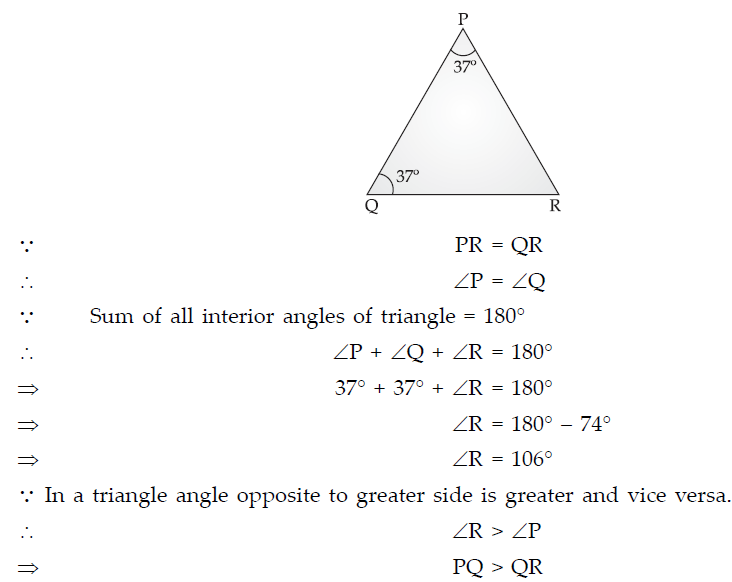(vii) (b) parallelogram

Explanation :

By mid-point theorem,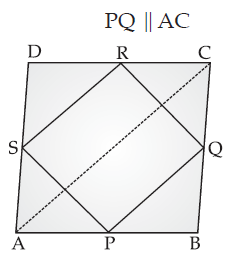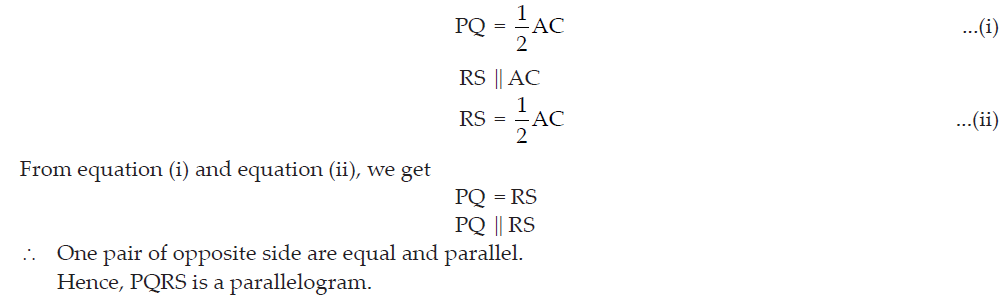(viii) (c) 40°

Explanation :

In the adjoining figure,
∠OAD = ∠OCB (Alternate angles)

∠OCB = 30°
∠AOB + ∠BOC = 180°
⇒ 70° + ∠BOC = 180°
⇒ ∠BOC = 180° – 70°
⇒ ∠BOC = 110°

In ΔBOC
∠OBC + ∠BOC + ∠OCB = 180°
⇒ ∠OBC + 110° + 30° = 180°
⇒ ∠OBC = 40°
⇒ ∠DBC = 40°

(ix) (b) 1 : 1

Explanation :

We know,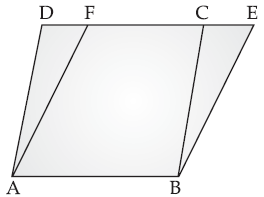Parallelograms on the same base and between the same parallel lines are equal in area (theorem).
Area of ABCD = Area of ABEF
Ratio = 1 : 1

(x) (b) False

Explanation :

Given, AD is diameter. AB can never be equal to AD because diameter is the largest chord of circle.
Hence AD > AB.Also, to make AB = AD, AD should pass through centre of circle.

(xi) (b) 16

Explanation :

Mean of 45 numbers = 18
⇒ Sum of 45 numbers = 18 × 45 = 810
Mean of (75 – 45) numbers = 13
Sum of 30 numbers = 13 × 30 = 390
Sum of 75 numbers = Sum of 45 numbers + Sum of 30 numbers.
Sum of 75 numbers = 810 + 390 = 1200

X=1200/75

=16

(xii) (b) 450 cm2
Explanation :

BC = 19 cmArea of quadrilateral ABCD = Area of ΔABC + Area of ΔBCD =1 x 30 x 11/2+1 x30x19/2=450 cm2

(xiii) (b) 70°

Explanation :

3 cot2 (x – 10°) = 1
⇒ cot2(x – 10°) = 1/3
⇒ cot(x – 10°) = 1/√3
We know, cot 60° = 1/√3
⇒ cot(x – 10°) = cot 60°
⇒ x – 10° = 60°
⇒ x = 70°

(xiv) (c) 10 √3 units

Explanation :

Draw perpendiculars on AB from DC.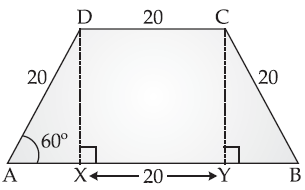In DDXA,
sin 60° = DX /DA
⇒ √3/2 = DX /20
⇒ DX = 10√3
⇒ Distance between AB and DC is 10√3 uunniittss.

(xv) (c) Scalene triangle

Explanation :• (i) We know that,
• (a + b)3 = a3 + b3 + 3a2b + 3ab2
• and (a – b)3 = a3 – b3 – 3a2b + 3ab2
• (a + b)3 – (a – b)3 = 2[b3 + 3a2b]
• (x/2+ y/3)3-(x/2 - y/3)3=2[(y/3)3+3[(y/3)3(y/3)]
• =2(y3/27+x2y/4)
• =2y3/27+x2y/2
• (ii) 5 – (3a2 – 2a) (6 – 3a2 + 2a) = 5 – (3a2 – 2a) [6 – (3a2 – 2a)]
• Let, 3a2 – 2a = x
• 5 – (3a2 – 2a) (6– 3a2 + 2a) = 5– x (6 – x) = 5 – 6x + x2
• = 5 – 5x – x + x2
• = 5(1 – x) – x (1 – x)
• = (5 – x) (1 – x)
• = (x – 5) (x – 1)
• = (3a2 – 2a – 5) (3a2 – 2a – 1) [Put x = 3a2 – 2a]
• = (3a2 – 5a + 3a – 5) (3a2 – 3a + a – 1)
• = {a (3a – 5) + 1 (3a – 5)} {3a (a – 1) + 1 (a – 1)}
• = (3a – 5) (a + 1) (3a + 1) (a – 1)
• (iii) Let the larger number be x and the smaller number be y.
• We know that,
• Dividend = Divisor × Quotient + Remainder
• 3x = (y × 4) + 3
• ⇒ 3x – 4y = 3 ...(i)
• and 7y = (x × 5) + 1
• ⇒ 5x – 7y = – 1 ...(ii)
• From equation (i), we have
• 3x = 4y + 3
• ⇒ x =4y=3/3 ...(iii)
• Substituting the value of x in equation (ii), we get
• 5((4y+3)/3)-7y=-1
• ⇒ 20y + 15 – 21y = – 3
• ⇒ – y = – 18
• ⇒ y = 18
• Putting the value of y in equation (iii), we get
• x =(4 x 18 + 3)/3=(72+3)/3=(75)/3=25
• x = 25 and y = 18
• The two numbers are 25 and 18. Ans.

• A = P(1+(r/100 x 2))2n
• ⇒ 4,576 = 4,400 (1+(8/100 x 2))2n
• ⇒ 4,576 = 4,400()26/25)2n
• ⇒ (26/25)2n=4,576/4,400
• ⇒ (26/25)2n=26/25
• ⇒ (1·04)2n = 1·04
• ⇒ (1·04)2n = (1·04)1
• From equation (i), we have
• ⇒ 2n = 1
• ⇒ n =1/2
• Thus, the required time period is half year. Ans.
• (ii) Given : AB = AC, BC = CD and DE || BC.
• (a) In ΔABC,
• AB = AC
• ⇒ ∠ACB = ∠ABC ...(i)
• Also, ∠ABC + ∠ACB = ∠FAB [Exterior angle property]
• ⇒ 2∠ABC = 128° [from equation (i)]
• ⇒ ∠ABC = 128@/2=64°
• ⇒ ∠ABC = ∠ACB = 64°
• InΔBCD,
• BC = CD [Given]
• ⇒ ∠BDC = ∠DBC ...(ii)
• Also, ∠BDC + ∠DBC + ∠BCD = 180° [Angle sum property]
• ⇒ 64° + 64° + ∠BCD = 180°
• ∠BCD = 180° – 128° = 52°
• Now, DE || BC
• ∠CDE = 52° Ans.
• (b) ∠ACB = ∠BCD + ∠DCE
• ⇒ ∠DCE = ∠ACB – ∠BCD
• = 64° – 52°
• ⇒ ∠DCE = 12°. Ans.
• (iii) We have,
• 5x – (5 – x) = 1/2 (3− x)
• ⇒ 5x – 5 + x = 1/2 (3− x)
• ⇒ 2(6x – 5) = 3 – x
• ⇒ 12x – 10 = 3 – x
• ⇒ 13x = 13
• x = 13 /13 =1
• Ans, 4 – 3y =(4y+y)/3
• ⇒ 3(4 – 3y) = 4 + y
• ⇒ 12 – 9y = 4 + y
• ⇒ – 9y – y = 4 – 12
• ⇒ – 10y = – 8
• ⇒ y =-18/-10=4/5
• So, the coordinates of the point are (1,4/5) Ans.

## Section-B

(i) Let us draw a right triangle ABC, right-angled at C in which tan A =1/√3• Now, tan A = BC/AC=1/√3
• Let BC = x
• and AC = √3 x
• By Pythagoras theorem, we
• AB2= AC2 + BC2
• ⇒ AB2 = (√ 3x)2 + x2
• ⇒ AB2 = 3x2 + x2
• ⇒ AB2 = 4x2
• ∴ AB = 2x
• With reference to ∠A, we have
• Base AC =√3x, Perpendicular BC=x and Hypotenuse AB=2x
• sin A = BC/AB = x/2x=1/2
• and, cosA =AC/AB
• =√3x/2x=√3/2
• With reference to ∠B, we have
• Base BC = x, Perpendicular AC = √3x and Hypotenuse AB = 2x.
• ∴ cos B =BC/AB=x/2x=1/2
• and sin B =AC/AB=√3x/2x=√3/2
• Now, sin A. cos B + cos A. sin B
• =1/2 x 1/2 + √3/2 x √3/2
• =1/4+3/4=1 Ans.
• (ii) ·.· Length of rectangular room = x
• ∴ Width = 4x/7
• ⇒ 2(x+4x/7)=y
• ⇒ 2(11x/7)=y
• ⇒ (22x/7)=y Ans.
• ∴ 22 x/7=4400
• ⇒ x = 1400
• Length of the room is 1400 cm or 14 m. Ans.
• (iii) (a) Class mark = ( Lower limit +Upper limit )/2
• =(90+120)/2=210/2=105 Ans.
• (b) Lower limit = Mid-value – Width of class/2 = 10-6/2
• = 10 – 3
• Lower limit of class = 7 Ans.
• (c) Given : Lower limit of the lowest class = 10
• Also, Width of each class = 5
• and Total number of classes = 5
• 1st class = 10 – 15, 2nd class = 15 – 20
• 3rd class = 20 – 25, 4th class = 25 – 30
• and 5th class = 30 – 35
• Upper limit of highest class = 35 Ans.

(i) Given : In DABC, AD is the median.
To prove : AB + AC > 2 AD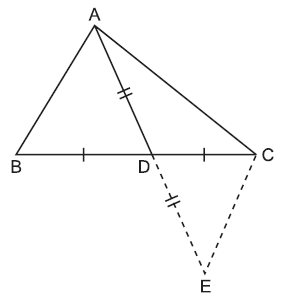• Construction : Produce AD upto a point E such that AD = DE and join C to E.
• Proof : In ΔACE,
• AC + CE > AE
• [... sum of two sides of a triangle is greater than the third side]
• ⇒ AC + CE > 2 AD [  AD = DE] ...(i)
• In ΔADB and ΔCDE,
• BD = CD [Given]
• AD = DE [By construction]
• ∠ADB = ∠CDE [Vertically opposite angle]
• ∠ADB ≅ ∠CDE [By SAS
• ∠ADB ≅ ∠CDE [By SAS congruencey rule]
• So, AB = CE [C.P.C.T.]So, AB = CE [C.P.C.T.]
• Now, substituting CE = AB in equation (i), we have
• AC + AB > 2 AD
• ⇒ AB + AC > 2 AD Hence Proved.
• (ii) Let us consider that √5 is a rational number.
• Then, let √5 =p/q, .....(i)
• where p and q are co-prime integers such that q ≠ 0, p and q have no common factor, except 1. Squaring on both sides in equation (i), we get
• ∴ 5 =p2/q2
• ⇒ p2 = 5q2 …(ii)
• ·.· 5q2 is divisible by 5.
• ∴ p2 is also divisible by 5.
• ∴ p is also divisible by 5.
• Let p = 5k, where k is an integer.
• ∴ p2 = 25k2
• ⇒ 5q2 = 25k2 [By using equation (ii)]
• ⇒ q2 = 5k2
• ·.· 5k2 is divisible by 5.
• ∴ q2 is also divisible by 5.
• ∴ q is also divisible by 5.
• ⇒ p and q both are divisible by 5.
• ⇒ p and q both multiples of 5.
• But p and q must have no common factor other than 1.
• It means our assumption is wrong.
• ∴ √5 is an irrational number. Hence Proved.
• (iii) Given : C is the mid-point of AB, ∠DCA = ∠ECB and ∠DBC = ∠EAC.
• To prove : DC = EC
• Proof : We have, ∠DCA = ∠ECB [Given]
• We have, ∠DCA + ∠DCE = ∠ECB + ∠DCE [Adding ∠DCE to both sides]
• ∠ACE = ∠DCB
• Now, in DACE and DDCB
• ∠ACE = ∠DCB [Proved above]
• AC = CB [Given]
• ∠CAE = ∠CBD [Given]
• By ASA congruency rule,
• ∠ACE ≅ DBCD
• DC = EC Hence Proved.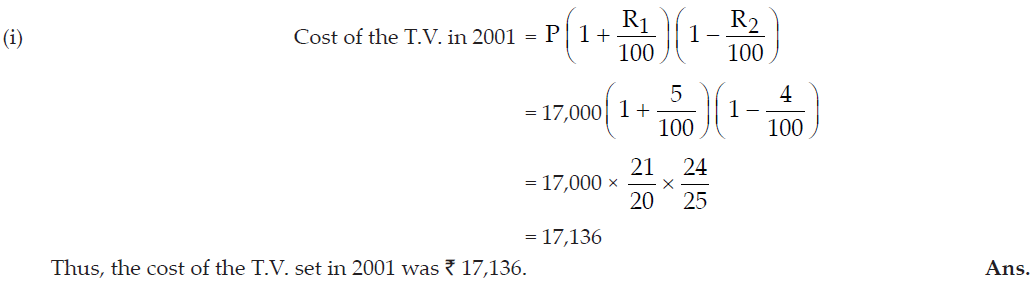• (ii) Given : PQ = PX,
• PR = PS
• and ∠QPX = ∠RPS
• To prove : QR = XS
• Proof : ∠QPX = ∠RPS
• ⇒ ∠QPX + ∠XPR = ∠RPS + ∠XPR [On adding ∠XPR both sides]
• ⇒ ∠QPR = ∠XPS ...(i)
• In DQPR and DXPS,
• PQ = PX [Given]
• ∠QPR = ∠XPS and PR = PS [Given]
• By SAS congruency rule
• DQPR ≅ DXPS
• QR = XS [C.P.C.T.] Hence Proved.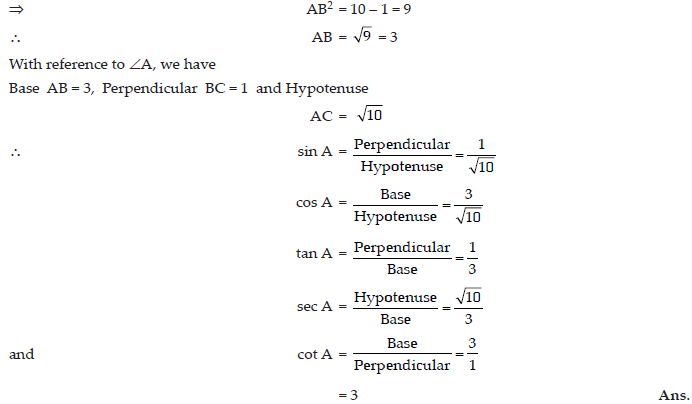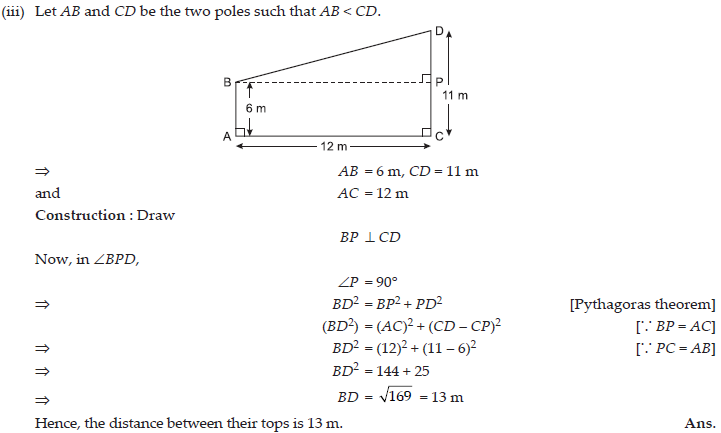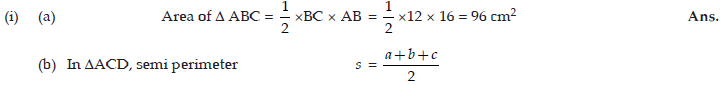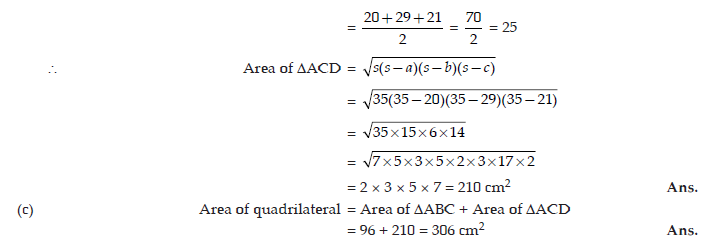• (ii) The given frequency distribution is in inclusive form, so first we convert it into exclusive form, as shown below.
 Height (in cm) Number of children (Frequency) 120.5—130.5 10 130.5—140.5 15 140.5—150.5 12 150.5—160.5 16 160.5—170.5 8 170.5—180.5 4• (i) Given, S.I. for 2 years = ₹ 200
• ⇒ P × 10 × 2/100=200
• ⇒ P = ₹ 1,000
• Now, C.I. for the first year
• = 1, 000 x 10 x 1/100=₹100
• ∴ Amount at the end of first year = `₹1,000 + ₹ 100 = ₹1,100
• C.I. for the second year =1,100 x 10 x1/100= ₹110
• ∴ Amount of the end of second year = ₹ 1,100 + ₹ 110 = ₹1,210
• So, Total C.I. in 2 years = ₹ 1,210 – ₹ 1,000 = ₹ 210. Ans.
• (ii) Given : ∠PRQ = 75°, ∠PSQ = 36° and PQ = PR
• ∠PRQ = ∠PQR = 75° [... PQ = QR]
• In ΔPQR,
• ∠PQR + ∠PRQ + ∠QPR = 180° [Angle sum property]
• ⇒ 75° + 75° + ∠QPR = 180°
• ⇒ ∠QPR = 180° – 150° = 30°
• ∠QPR < ∠PQR
• QR < PR ...(i)
• [ Side opposite to smaller angle is shorter]
• ∠RPS + ∠PSR = ∠PRQ [Exterior angle property]
• ⇒ ∠RPS + 36° = 75°
• ⇒ ∠RPS = 75° – 36° = 39°
• ∠PSR < ∠RPS
• PR < RS ...(ii)
• From equation (i) and equation (ii), we get
• QR < PR < RS Ans.
• (iii) Let O (x, y) be the centre of the circle.
• Also, let A (6, – 6), B (3, – 7), C (3, 3) be the three points which lie on the circle.• ⇒ (6 – x)2 + (– 6 – y)2 = (3 – x)2 + (– 7 – y)2
• ⇒ 36 + x2 – 12x + 36 + y2 + 12y = 9 + x2 – 6x + 49 + y2 + 14y
• ⇒ – 12x + 6x + 12y – 14y + 72 – 58 = 0
• ⇒ – 6x – 2y + 14 = 0
• ⇒ – 2 (3x + y – 7) = 0
• ⇒ 3x + y = 7 ...(i)
• Similarly, OA = OC
• or OA2 = OC2
• ⇒ (6 – x )2 + (– 6 – y)2 = (3 – x)2 + (3 – y)2
• ⇒ 36 + x2 – 12x + 36 + y2 + 12y = 9 + x2 – 6x + 9 + y2 – 6y
• ⇒ – 12x + 6x + 12y + 6y + 72 – 18 = 0
• ⇒ – 6x + 18y + 54 = 0
• ⇒ – 6 (x – 3y – 9) = 0
• ⇒ x – 3y = 9 ...(ii)
• Solving equations (i) and (ii), we get
• x = 3 y = – 2
• Hence, the centre of circle is (3, – 2). Ans.

#### ICSE 61 Sample Question Papers

All Subjects Combined for Class 9 Exam 2023

The dot mark field are mandatory, So please fill them in carefully
To download the Sample Paper (PDF File), Please fill & submit the form below.

Free Shipping on all
orderes over ₹ 1,000

Free 10-Day
Return Policy

15-Days Money-back
Guarantee

23,125 Products
Shipped this Month

Need Help?
+91-745 507 7222

Shopping cart